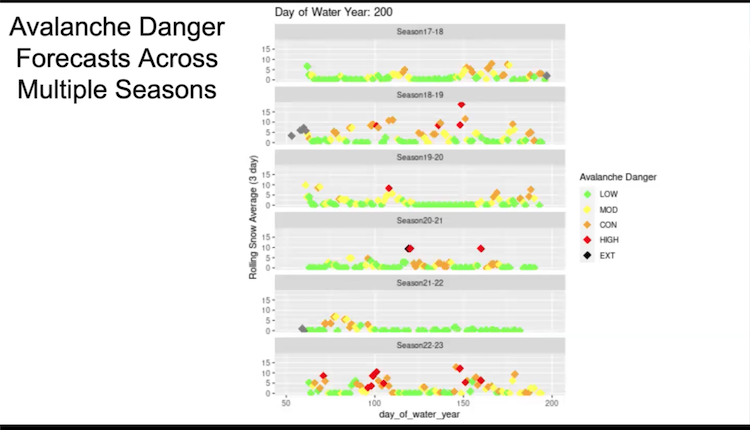# Simple Random Sampling: Not So Simple

Published:

This blog was originally posted on the BIDS blog, here.

Simple random sampling is drawing \$k\$ objects from a group of \$n\$ in such a way that all \${n\choose k}\$ possible subsets are equally likely. In practice, it is difficult to draw truly random samples. Instead, people tend to draw samples using

1. A pseudorandom number generator (PRNG) that produces sequences of bits, plus
2. A sampling algorithm that maps a sequence of pseudorandom numbers into a subset of the population.

Most people take for granted that this procedure is a sufficient approximation to simple random sampling. If it isn’t, then many statistical results may be called into question: anything that relies on sampling, including permutations, bootstrapping, and Monte Carlo simulations, may give biased results.

This blog post is a preview of what I plan to talk about at the UC Berkeley Risk Management Seminar on Tuesday, February 7. This is joint work with Philip B. Stark and Ronald Rivest.

## Finite state space

A PRNG is a deterministic function with several components:

• A user-supplied seed value used to set the internal state
• A function that maps the internal state to pseudorandom bits
• A function that updates the internal stateThe internal state of a PRNG is usually stored as an integer or matrix with fixed size. As such, it can only take on finitely many values. PRNGs are periodic: if we generate enough pseudorandom numbers, we will update the internal state so many times that the PRNG will return to its starting state.

This periodicity is a problem. PRNGs are deterministic, so for each value of the internal state, our sampling algorithm of choice will give us exactly one random sample. If the number of samples of size \$k\$ from a population of \$n\$ is greater than the size of the PRNG’s state space, then the PRNG cannot possibly generate all samples.

This will certainly be a problem for most PRNGs when \$n\$ and \$k\$ grow large, even for those like the Mersenne Twister, which is widely accepted and used as the default PRNG in most common software packages.

## Cryptographically secure PRNGs

One solution is to use PRNGs that have an infinite state space. Cryptographers have worked extensively on this problem, but cryptographically secure PRNGs haven’t yet become mainstream in other fields. They’re a bit slower than the PRNGs in wide use, so they’re typically reserved for applications where security is important. For the purpose of sampling, the bulk of the computational time will be spent in the sampling algorithm and not in the PRNG, so we are less concerned.

Hash functions take in a message of arbitrary length and return a hashed value of fixed length (e.g. 256 bits). A cryptographic hash function has the additional properties that it is computationally infeasible to invert in polynomial time; it’s difficult to find two inputs that hash to the same output; small changes to the input message produce large, unpredictable changes to the output; and the output bits are uniformly distributed. These properties are amenable to generating pseudorandom numbers. The diagram gives a cartoon of how a hash function operates on a message \$x\$ to output a hashed value \$h(x)\$.We are developing plug-in PRNGs based on the SHA256 hash function for R and Python. The Python package is in development on GitHub. The code is currently only prototyped in Python, but watch our repository for a sped up C implementation.

## Tests for pseudorandomness

Generating pseudorandom numbers with traditional PRNGs is a problem when \$n\$ and \$k\$ grow large, but how do they perform for small or moderate \$n\$ and \$k\$? I would argue that if we’re using PRNGs for statistical methods, we should judge their performance by how well they can generate simple random samples. We are actively working on testing PRNGs for this goal and hope to have a paper out later this year. Stay tuned!